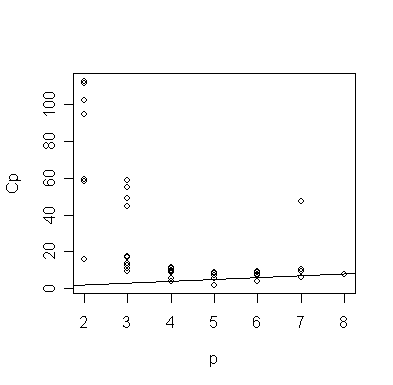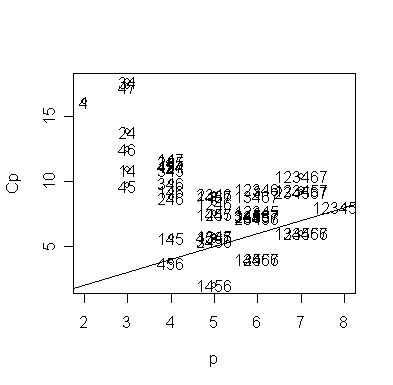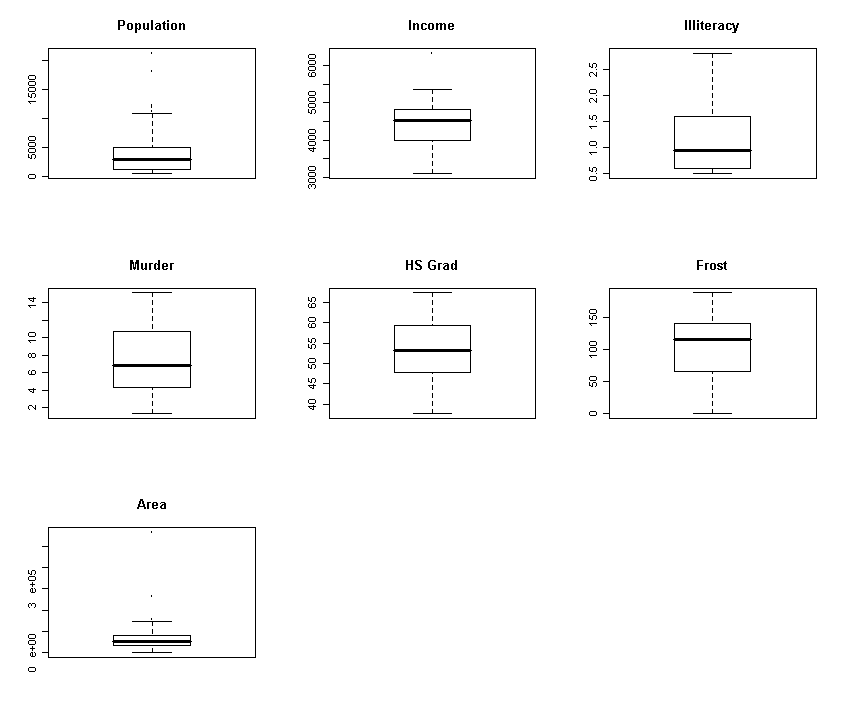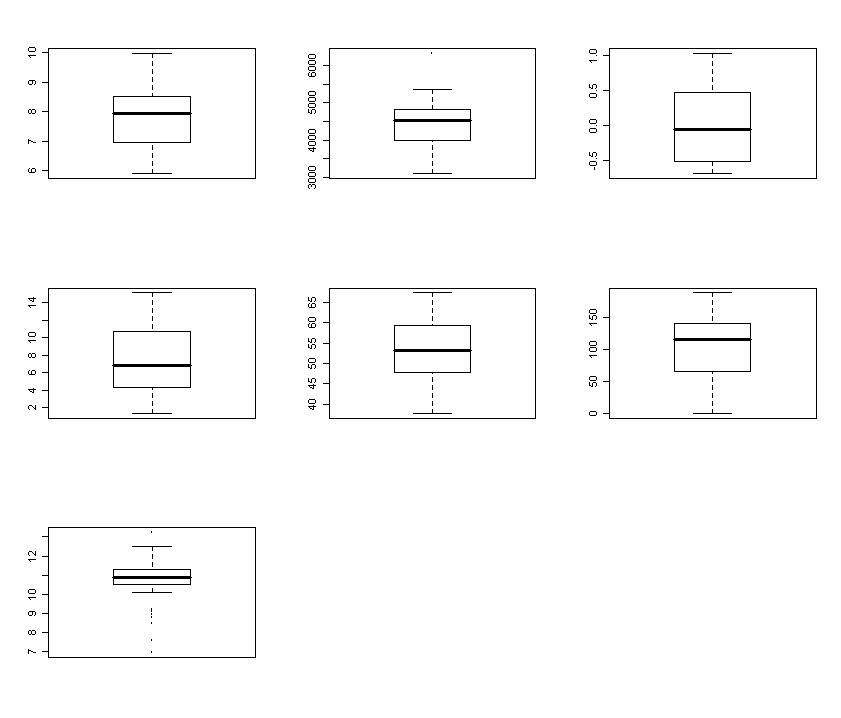# Model (variable) selection

Tesging-based procedure (Reading: Faraway (2005, 1st edition), 8.2)

We illustrate the variable selection methods on a build-in dataset (called "state") in R, which contains some data on the 50 states (use the command "help(state)" to get more information). We will use the data matrix "state.x77" which gives the population estimate as of july 1, 1975; per capita income (1974); illiteracy (1970, percent of population); life expectancy in years (1969-71); murder and non-negligent manslaughter rate per 100,000 population (1976); percent high-school graduates (1970); mean number of days with min temperature < 32 degrees (1931-1960) in capital or large city; and land area in square miles. We will take life expectancy as the response and the remaining variables as predictors - we extract these for convenience and fit the full model.

> data(state)

> statedata <- data.frame(state.x77, row.names=state.abb, check.names=T)

> statedata # take a look

Population Income Illiteracy Life.Exp Murder HS.Grad Frost   Area

AL       3615   3624        2.1    69.05   15.1    41.3    20  50708

AK        365   6315        1.5    69.31   11.3    66.7   152 566432

AZ       2212   4530        1.8    70.55    7.8    58.1    15 113417

… deleted …

WY        376   4566        0.6    70.29    6.9    62.9   173  97203

> g <- lm(Life.Exp ~ ., data=statedata)

> summary(g)

Coefficients:

Estimate Std. Error t value Pr(>|t|)

(Intercept)  7.094e+01  1.748e+00  40.586  < 2e-16 ***

Population   5.180e-05  2.919e-05   1.775   0.0832 .

Income      -2.180e-05  2.444e-04  -0.089   0.9293

Illiteracy   3.382e-02  3.663e-01   0.092   0.9269

Murder      -3.011e-01  4.662e-02  -6.459 8.68e-08 ***

HS.Grad      4.893e-02  2.332e-02   2.098   0.0420 *

Frost       -5.735e-03  3.143e-03  -1.825   0.0752 .

Area        -7.383e-08  1.668e-06  -0.044   0.9649

---

Signif. codes:  0 `***' 0.001 `**' 0.01 `*' 0.05 `.' 0.1 ` ' 1

Residual standard error: 0.7448 on 42 degrees of freedom

Multiple R-Squared: 0.7362,     Adjusted R-squared: 0.6922

F-statistic: 16.74 on 7 and 42 DF,  p-value: 2.534e-10

Which predictors should be included - can you tell from the p-values? Looking at the coefficients, can you see what operation would be helpful? Does the murder rate decrease life expectancy - that's obvious a priori but how should these results be interpreted?

We illustrate the backward method --- at each step, we remove the predictor with the largest p-value over 0.05. [Note: In R, the function "mle.stepwise()" in "wle" library can automatically do the job. See the "help(mle.stepwise)" page in R for details.]

> g <- update(g, .~. - Area); summary(g)

Call:

lm(formula = Life.Exp ~ Population + Income + Illiteracy + Murder +

HS.Grad + Frost, data = statedata)

Residuals:

Min       1Q   Median       3Q      Max

-1.49047 -0.52533 -0.02546  0.57160  1.50374

Coefficients:

Estimate Std. Error t value Pr(>|t|)

(Intercept)  7.099e+01  1.387e+00  51.165  < 2e-16 ***

Population   5.188e-05  2.879e-05   1.802   0.0785 .

Income      -2.444e-05  2.343e-04  -0.104   0.9174

Illiteracy   2.846e-02  3.416e-01   0.083   0.9340

Murder      -3.018e-01  4.334e-02  -6.963 1.45e-08 ***

HS.Grad      4.847e-02  2.067e-02   2.345   0.0237 *

Frost       -5.776e-03  2.970e-03  -1.945   0.0584 .

---

Signif. codes:  0 '***' 0.001 '**' 0.01 '*' 0.05 '.' 0.1 ' ' 1

Residual standard error: 0.7361 on 43 degrees of freedom

Multiple R-Squared: 0.7361,     Adjusted R-squared: 0.6993

F-statistic: 19.99 on 6 and 43 DF,  p-value: 5.362e-11

> g <- update(g, .~. - Illiteracy); summary(g)

Call:

lm(formula = Life.Exp ~ Population + Income + Murder + HS.Grad +

Frost, data = statedata)

Residuals:

Min       1Q   Median       3Q      Max

-1.48921 -0.51224 -0.03290  0.56447  1.51662

Coefficients:

Estimate Std. Error t value Pr(>|t|)

(Intercept)  7.107e+01  1.029e+00  69.067  < 2e-16 ***

Population   5.115e-05  2.709e-05   1.888   0.0657 .

Income      -2.477e-05  2.316e-04  -0.107   0.9153

Murder      -3.000e-01  3.704e-02  -8.099 2.91e-10 ***

HS.Grad      4.776e-02  1.859e-02   2.569   0.0137 *

Frost       -5.910e-03  2.468e-03  -2.395   0.0210 *

---

Signif. codes:  0 '***' 0.001 '**' 0.01 '*' 0.05 '.' 0.1 ' ' 1

Residual standard error: 0.7277 on 44 degrees of freedom

Multiple R-Squared: 0.7361,     Adjusted R-squared: 0.7061

F-statistic: 24.55 on 5 and 44 DF,  p-value: 1.019e-11

> g <- update(g, .~. - Income); summary(g)

Call:

lm(formula = Life.Exp ~ Population + Murder + HS.Grad + Frost,

data = statedata)

Residuals:

Min       1Q   Median       3Q      Max

-1.47095 -0.53464 -0.03701  0.57621  1.50683

Coefficients:

Estimate Std. Error t value Pr(>|t|)

(Intercept)  7.103e+01  9.529e-01  74.542  < 2e-16 ***

Population   5.014e-05  2.512e-05   1.996  0.05201 .

Murder      -3.001e-01  3.661e-02  -8.199 1.77e-10 ***

HS.Grad      4.658e-02  1.483e-02   3.142  0.00297 **

Frost       -5.943e-03  2.421e-03  -2.455  0.01802 *

---

Signif. codes:  0 '***' 0.001 '**' 0.01 '*' 0.05 '.' 0.1 ' ' 1

Residual standard error: 0.7197 on 45 degrees of freedom

Multiple R-Squared: 0.736,      Adjusted R-squared: 0.7126

F-statistic: 31.37 on 4 and 45 DF,  p-value: 1.696e-12

> g <- update(g, .~. - Population); summary(g)

Call:

lm(formula = Life.Exp ~ Murder + HS.Grad + Frost, data = statedata)

Residuals:

Min      1Q  Median      3Q     Max

-1.5015 -0.5391  0.1014  0.5921  1.2268

Coefficients:

Estimate Std. Error t value Pr(>|t|)

(Intercept) 71.036379   0.983262  72.246  < 2e-16 ***

Murder      -0.283065   0.036731  -7.706 8.04e-10 ***

HS.Grad      0.049949   0.015201   3.286  0.00195 **

Frost       -0.006912   0.002447  -2.824  0.00699 **

---

Signif. codes:  0 '***' 0.001 '**' 0.01 '*' 0.05 '.' 0.1 ' ' 1

Residual standard error: 0.7427 on 46 degrees of freedom

Multiple R-Squared: 0.7127,     Adjusted R-squared: 0.6939

F-statistic: 38.03 on 3 and 46 DF,  p-value: 1.634e-12

The final removal of the Population variable is a close call. We may want to consider including this variable if interpretation is aided. Notice that the R2 for the full model of 0.736 is reduced only slightly to 0.713 in the final model. Thus the removal of four predictors causes only a minor reduction in fit (Note the adjusted R2 increases).

It is important to understand that the variables omitted from the model may still be related to the response. For example, let us fit a model with Illiteracy, Murder, and Frost as predictors:

> summary(lm(Life.Exp~Illiteracy+Murder+Frost, statedata))

Call:

lm(formula = Life.Exp ~ Illiteracy + Murder + Frost, data = statedata)

Residuals:

Min        1Q    Median        3Q       Max

-1.590098 -0.469608  0.003935  0.570604  1.922922

Coefficients:

Estimate Std. Error t value Pr(>|t|)

(Intercept) 74.556717   0.584251 127.611  < 2e-16 ***

Illiteracy  -0.601761   0.298927  -2.013  0.04998 *

Murder      -0.280047   0.043394  -6.454 6.03e-08 ***

Frost       -0.008691   0.002959  -2.937  0.00517 **

---

Signif. codes:  0 '***' 0.001 '**' 0.01 '*' 0.05 '.' 0.1 ' ' 1

Residual standard error: 0.7911 on 46 degrees of freedom

Multiple R-Squared: 0.6739,     Adjusted R-squared: 0.6527

F-statistic: 31.69 on 3 and 46 DF,  p-value: 2.915e-11

We can see that Illiteracy does have some association with Life Expectancy although Illiteracy was removed during the backward selection. It is true that replacing Illiteracy with High School graduation rate gave us a somewhat better fitted model in terms of R2. But, it would be insufficient to conclude that Illiteracy is not a variable of interest. This example also shows one drawback of testing-based procedure: when there are several models that can fit the data equally well, the testing-based procedure ends up with only one model and offers no information about other good fitted models.

Criterion-based procedure (Reading: Faraway (2005, 1st edition), 8.3)

Now, let's try some criterion-based methods. Let's start from the AIC. In R, AIC for model selection is implemented with a stepwise procedure in the function "step()" or "stepAIC()". The function does not evaluate the AIC for all possible models but uses a search method ("forward", "backward", or "both", default is "both") that compares models sequentially. Thus it bears some comparison to the stepwise method described above but with the advantage that no dubious p-values are used.

> g <- lm(Life.Exp ~ ., data=statedata)

> step(g)

Start:  AIC= -22.18

Life.Exp ~ Population + Income + Illiteracy + Murder + HS.Grad + Frost + Area

Df Sum of Sq     RSS     AIC

- Area        1     0.001  23.298 -24.182

- Income      1     0.004  23.302 -24.175

- Illiteracy  1     0.005  23.302 -24.174

<none>                     23.297 -22.185

- Population  1     1.747  25.044 -20.569

- Frost       1     1.847  25.144 -20.371

- HS.Grad     1     2.441  25.738 -19.202

- Murder      1    23.141  46.438  10.305

Step:  AIC= -24.18

Life.Exp ~ Population + Income + Illiteracy + Murder + HS.Grad + Frost

Df Sum of Sq     RSS     AIC

- Illiteracy  1     0.004  23.302 -26.174

- Income      1     0.006  23.304 -26.170

<none>                     23.298 -24.182

- Population  1     1.760  25.058 -22.541

- Frost       1     2.049  25.347 -21.968

- HS.Grad     1     2.980  26.279 -20.163

- Murder      1    26.272  49.570  11.568

Step:  AIC= -26.17

Life.Exp ~ Population + Income + Murder + HS.Grad + Frost

Df Sum of Sq     RSS     AIC

- Income      1     0.006  23.308 -28.161

<none>                     23.302 -26.174

- Population  1     1.887  25.189 -24.280

- Frost       1     3.037  26.339 -22.048

- HS.Grad     1     3.495  26.797 -21.187

- Murder      1    34.739  58.041  17.457

Step:  AIC= -28.16

Life.Exp ~ Population + Murder + HS.Grad + Frost

Df Sum of Sq     RSS     AIC

<none>                     23.308 -28.161

- Population  1     2.064  25.372 -25.920

- Frost       1     3.122  26.430 -23.876

- HS.Grad     1     5.112  28.420 -20.246

- Murder      1    34.816  58.124  15.528

Call:

lm(formula = Life.Exp ~ Population + Murder + HS.Grad + Frost, data = statedata)

Coefficients:

7.103e+01    5.014e-05   -3.001e-01    4.658e-02   -5.943e-03

The sequence of variable removal is the same as with backward elimination. The only difference is the Population variable is retained.

Now we try the Cp and adjusted R2 methods for the selection of variables in the State dataset. The function "leaps()" supports the Mallow's Cp and adjusted R2 criteria. The default for the function is the Mallow's Cp criterion:

> library(leaps)

> x <- statedata[,-4]
> y <- statedata[,4]
> gcp <- leaps(x,y)
> gcp

\$which

1     2     3     4     5     6     7

1 FALSE FALSE FALSE  TRUE FALSE FALSE FALSE

1 FALSE FALSE  TRUE FALSE FALSE FALSE FALSE

1 FALSE FALSE FALSE FALSE  TRUE FALSE FALSE

1 FALSE  TRUE FALSE FALSE FALSE FALSE FALSE

1 FALSE FALSE FALSE FALSE FALSE  TRUE FALSE

1 FALSE FALSE FALSE FALSE FALSE FALSE  TRUE

1  TRUE FALSE FALSE FALSE FALSE FALSE FALSE

2 FALSE FALSE FALSE  TRUE  TRUE FALSE FALSE

2  TRUE FALSE FALSE  TRUE FALSE FALSE FALSE

2 FALSE FALSE FALSE  TRUE FALSE  TRUE FALSE

2 FALSE  TRUE FALSE  TRUE FALSE FALSE FALSE

2 FALSE FALSE FALSE  TRUE FALSE FALSE  TRUE

2 FALSE FALSE  TRUE  TRUE FALSE FALSE FALSE

2 FALSE FALSE FALSE FALSE  TRUE FALSE  TRUE

2 FALSE FALSE  TRUE FALSE  TRUE FALSE FALSE

2 FALSE FALSE  TRUE FALSE FALSE  TRUE FALSE

2 FALSE  TRUE  TRUE FALSE FALSE FALSE FALSE

3 FALSE FALSE FALSE  TRUE  TRUE  TRUE FALSE

3  TRUE FALSE FALSE  TRUE  TRUE FALSE FALSE

3 FALSE  TRUE FALSE  TRUE FALSE  TRUE FALSE

3  TRUE FALSE FALSE  TRUE FALSE  TRUE FALSE

3 FALSE FALSE  TRUE  TRUE FALSE  TRUE FALSE

3 FALSE FALSE  TRUE  TRUE  TRUE FALSE FALSE

3 FALSE FALSE FALSE  TRUE  TRUE FALSE  TRUE

3  TRUE  TRUE FALSE  TRUE FALSE FALSE FALSE

3 FALSE  TRUE FALSE  TRUE  TRUE FALSE FALSE

3  TRUE FALSE FALSE  TRUE FALSE FALSE  TRUE

4  TRUE FALSE FALSE  TRUE  TRUE  TRUE FALSE

4 FALSE  TRUE FALSE  TRUE  TRUE  TRUE FALSE

4 FALSE FALSE  TRUE  TRUE  TRUE  TRUE FALSE

4 FALSE FALSE FALSE  TRUE  TRUE  TRUE  TRUE

4  TRUE FALSE  TRUE  TRUE  TRUE FALSE FALSE

4  TRUE FALSE FALSE  TRUE  TRUE FALSE  TRUE

4  TRUE  TRUE FALSE  TRUE  TRUE FALSE FALSE

4  TRUE  TRUE FALSE  TRUE FALSE  TRUE FALSE

4  TRUE FALSE FALSE  TRUE FALSE  TRUE  TRUE

4 FALSE  TRUE  TRUE  TRUE FALSE  TRUE FALSE

5  TRUE  TRUE FALSE  TRUE  TRUE  TRUE FALSE

5  TRUE FALSE  TRUE  TRUE  TRUE  TRUE FALSE

5  TRUE FALSE FALSE  TRUE  TRUE  TRUE  TRUE

5 FALSE  TRUE  TRUE  TRUE  TRUE  TRUE FALSE

5 FALSE  TRUE FALSE  TRUE  TRUE  TRUE  TRUE

5  TRUE FALSE  TRUE  TRUE  TRUE FALSE  TRUE

5 FALSE FALSE  TRUE  TRUE  TRUE  TRUE  TRUE

5  TRUE  TRUE  TRUE  TRUE  TRUE FALSE FALSE

5  TRUE FALSE  TRUE  TRUE FALSE  TRUE  TRUE

5  TRUE  TRUE  TRUE  TRUE FALSE  TRUE FALSE

6  TRUE  TRUE  TRUE  TRUE  TRUE  TRUE FALSE

6  TRUE FALSE  TRUE  TRUE  TRUE  TRUE  TRUE

6  TRUE  TRUE FALSE  TRUE  TRUE  TRUE  TRUE

6 FALSE  TRUE  TRUE  TRUE  TRUE  TRUE  TRUE

6  TRUE  TRUE  TRUE  TRUE  TRUE FALSE  TRUE

6  TRUE  TRUE  TRUE  TRUE FALSE  TRUE  TRUE

6  TRUE  TRUE  TRUE FALSE  TRUE  TRUE  TRUE

7  TRUE  TRUE  TRUE  TRUE  TRUE  TRUE  TRUE

\$label

 "(Intercept)" "1"           "2"           "3"           "4"

 "5"           "6"           "7"

\$size

 2 2 2 2 2 2 2 3 3 3 3 3 3 3 3 3 3 4 4 4 4 4 4 4 4 4 4 5 5 5 5 5 5 5 5 5 5 6 6 6

 6 6 6 6 6 6 6 7 7 7 7 7 7 7 8

\$Cp

  16.126760  58.058325  59.225241  94.755684 102.252357 111.351299 112.447938

   9.669894  10.886508  12.475673  13.791499  17.280014  17.634184  44.940876

  49.351549  54.896851  58.701223   3.739878   5.647595   8.727618   9.236254

   9.903176  10.873258  11.169557  11.190554  11.485646  11.705240   2.019659

   5.411184   5.430093   5.693495   5.780351   7.482910   7.484304   8.309352

   8.884194   9.015565   4.008737   4.012588   4.018235   7.174687   7.306932

   7.344256   7.428952   7.695471   8.888310   9.375028   6.001959   6.007958

   6.008526   9.149820   9.329008  10.401108  47.718647   8.000000

You may observe that not all sub-models are reported (only 55 models in this case). The number of reported best models is controlled by the option "nbest" in "leaps" function.

It's usual to plot the Cp data against p:

> plot(gcp\$size, gcp\$Cp, xlab="p", ylab="Cp"); abline(0,1)The plot is distorted by the large Cp values - let's exclude them:

> small <- (gcp\$Cp < 20) # logical vector of Cp values smaller than 20

> plot(gcp\$size[small], gcp\$Cp[small], xlab="p", ylab="Cp"); abline(0,1) # plot Cp against p for Cp < 20

> gcp.labels <- apply(gcp\$which,1,function(x) paste(as.character((1:7)[x]),collapse="")) # generate model labels

> text(gcp\$size[small], gcp\$Cp[small], gcp.labels[small]) # add the model labelsThe models are denoted by indices for the predictors. The competition is between the "456" model, i.e., the Forest, HS graduation, and Murder model, and the model also including Population. Both models are on or below the Cp=p line, indicating good fits. The choice is between the smaller model and the larger model which fits a little better. Some even larger models fit in the sense that they are on or below the Cp=p line but we would not opt for these in the presence of smaller models that fit.

Now let's see which model the adjusted R2 criterion selects.

> gadjr # take a look

Let's look at the 10 best (in the sense of having high adjusted R2):

1456   12456   13456   14567  123456  134567  124567     456 1234567    2456

0.713   0.706   0.706   0.706   0.699   0.699   0.699   0.694   0.692   0.689

How does this compare to previous results? We can see that the model with Population, Frost, HS graduation, and Murder model has the largest adjusted R2. The best three predictor model picked by Cp is in 8th place but the intervening models are not attractive since they use more predictors than the best model.

Variable selection methods are sensitive to outliers and influential points. Let's check for high leverage points:

> h <- hat(x)
> names(h) <- state.abb

> rev(sort(h))[1:8]

AK        CA        HI        NV        NM        TX        NY        WA

0.8095223 0.4088569 0.3787617 0.3652458 0.3247216 0.2841635 0.2569498 0.2226820

Which state sticks out? Let's try excluding it (Alaska is the second state in the data):

> l.labels <- apply(l\$which,1,function(x) paste(as.character((1:7)[x]),collapse=""))

> i <- order(l\$adjr2, decreasing = T)

14567    1456  124567   24567  134567   13456   12456 1234567  234567    4567

0.710   0.709   0.707   0.707   0.704   0.703   0.703   0.701   0.700   0.700

Compare with the "best" model selected above. We see that Area now makes it into the model.

Transforming the predictors can also have an effect. Let's take a look at the variables:

> par(mfrow=c(3,3))

> for (i in c(1:3, 5:8)) boxplot(state.x77[,i], main=dimnames(state.x77)[][i])We see that Population, Illiteracy and Area are skewed - we try transforming them:

> nx <- cbind(log(x[,1]),x[,2],log(x[,3]),x[,4:6],log(x[,7]))

And now replot:

> par(mfrow=c(3,3))

> apply(nx,2,boxplot)It shows the appropriately transformed data.

Now try the adjusted R2 method again - we make sure the columns of the new matrix have the right label names for ease of reference.